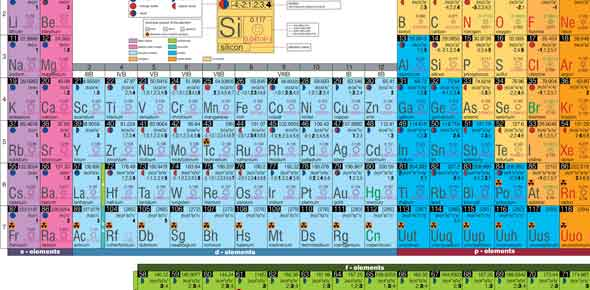# Periodic Table - Trends & Families

20 Questions | Attempts: 1125
ShareSettingsElements said to be in the same family if they have similarities and for instance boron and lithium are in the same family because they got the largest atomic sizes. What else do you know about the families in which the different elements exist? Take up the quiz and get to find out.

• 1.
Ionization energy refers to
• A.

The energy needed to excite an electron

• B.

The energy needed to remove an electron

• C.

The energy needed to attract an electron

• D.

The energy needed to release a photon

• 2.
Electronegativity refers to
• A.

The energy needed to excite an electron

• B.

The energy needed to remove an electron

• C.

The energy needed to attract an electron

• D.

The energy needed to release a photon

• 3.
The alkali metals family has _______ valence electrons.
• A.

1

• B.

2

• C.

6

• D.

7

• E.

8

• 4.
Magneiusm is in the __________ family.
• A.

Alkali metal

• B.

Alkaline earth metal

• C.

Transition metal

• D.

Chalcogen

• E.

Halogen

• 5.
Which of the following elements is an alkali metal?
• A.

Hydrogen, H

• B.

Lithium, Li

• C.

Beryllium, Be

• D.

• 6.
Which element in period 5 has the largest atomic radii?
• A.

Rubidium, Rb

• B.

Xenon, Xe

• C.

• D.

Duborium, Db

• 7.
Which element in group 16 has the largest atomic radius?
• A.

Oxygen, O

• B.

Sulfur, S

• C.

Selenium, Se

• D.

Tellurium, Te

• E.

Polonium, Po

• F.

Ununhexium, Uuh

• 8.
• A.

Alkali metals

• B.

Transition metals

• C.

Lanthanides

• D.

Actinides

• 9.
Which family is the least reactive?
• A.

Alkali metals

• B.

Chalcogens

• C.

Transition metalsals

• D.

Noble gases

• 10.
How many valence electrons does Helium (He) have?
• A.

1

• B.

2

• C.

7

• D.

8

• 11.
Why are halogens so highly reactive?
• A.

They have 1 valence electron and want to get rid of it

• B.

They have 1 valence electrons and want 7 more electrons

• C.

They have 7 valence electrons and want 1 more electron

• D.

They have 7 valence electrons and want to get rid of all 7 electrons

• 12.
What is the same within a period?
• A.

The number of valence electrons

• B.

The number of orbits/shells

• C.

The reactivity characteristics

• D.

The physical properties (color, melting point, boiling point)

• 13.
The most electronegative element on the periodic table is
• A.

Lithium, Li

• B.

Francium, Fr

• C.

Astatine, At

• D.

Fluorine, F

• 14.
Iron, Fe is smaller than Hassium (Hs), primarily because
• A.

Iron has fewer neutrons

• B.

Iron has a smaller mass

• C.

Iron has a smaller atomic number

• D.

Iron has fewer orbits

• 15.
The element with the lowest ionization energy  periodic table is
• A.

Lithium, Li

• B.

Francium, Fr

• C.

Astatine, At

• D.

Fluorine, F

• 16.
Electronegativity __________ as you go down a column because the electrons are ________ to/from the nucleus.
• A.

Increases, closer

• B.

Decreases, closer

• C.

Increases, farther

• D.

Decreases, farther

• 17.
The most electronegative element on the periodic table is
• A.

Lithium, Li

• B.

Francium, Fr

• C.

Astatine, At

• D.

Fluorine, F

• 18.
Atomic radius __________ as you go across a period because ________
• A.

Increases, there are more orbits

• B.

Increases, the nucleus pulls less on the electrons

• C.

Decreases, there are less orbits

• D.

Decreases, the nucleus pulls more on the electrons

• 19.
Transition metals have _________ valence electrons.
• A.

1

• B.

2

• C.

6

• D.

8

• 20.
_______ is an example of a chalcogen
• A.

Lithium, Li

• B.

Barium, Ba

• C.

Selenium, Se

• D.

Neon, Ne

## Related TopicsBack to top
×

Wait!
Here's an interesting quiz for you.